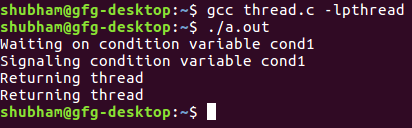GFG App
Open AppBrowser
Continue

# Conditional wait and signal in multi-threading

What are conditional wait and signal in multi-threading?
Explanation: When you want to sleep a thread, condition variable can be used. In C under Linux, there is a function pthread_cond_wait() to wait or sleep.
On the other hand, there is a function pthread_cond_signal() to wake up sleeping or waiting thread.
Threads can wait on a condition variable.

```int pthread_cond_wait(pthread_cond_t *restrict cond,

Parameter :

```cond : condition variable
mutex : is mutex lock ```

Return Value :

```On success, 0 is returned ; otherwise, an error
number shall be returned to indicate the error. ```

The pthread_cond_wait() release a lock specified by mutex and wait on condition cond variable.

`int pthread_cond_signal(pthread_cond_t *cond);`

Parameter :

`cond : condition variable`

Return Value :

```On success, 0 is returned ; otherwise, an error number
shall be returned to indicate the error. ```

Note : The above two functions works together.

Below is the implementation of condition, wait and signal functions.

## C

 `// C program to implement cond(), signal()` `// and wait() functions` `#include ` `#include ` `#include `   `// Declaration of thread condition variable` `pthread_cond_t cond1 = PTHREAD_COND_INITIALIZER;`   `// declaring mutex` `pthread_mutex_t lock = PTHREAD_MUTEX_INITIALIZER;`   `int` `done = 1;`   `// Thread function` `void``* foo()` `{`   `    ``// acquire a lock` `    ``pthread_mutex_lock(&lock);` `    ``if` `(done == 1) {`   `        ``// let's wait on condition variable cond1` `        ``done = 2;` `        ``printf``(``"Waiting on condition variable cond1\n"``);` `        ``pthread_cond_wait(&cond1, &lock);` `    ``}` `    ``else` `{`   `        ``// Let's signal condition variable cond1` `        ``printf``(``"Signaling condition variable cond1\n"``);` `        ``pthread_cond_signal(&cond1);` `    ``}`   `    ``// release lock` `    ``pthread_mutex_unlock(&lock);`   `    ``printf``(``"Returning thread\n"``);`   `    ``return` `NULL;` `}`   `// Driver code` `int` `main()` `{` `    ``pthread_t tid1, tid2;`   `    ``// Create thread 1` `    ``pthread_create(&tid1, NULL, foo, NULL);`   `    ``// sleep for 1 sec so that thread 1` `    ``// would get a chance to run first` `    ``sleep(1);`   `    ``// Create thread 2` `    ``pthread_create(&tid2, NULL, foo, NULL);`   `    ``// wait for the completion of thread 2` `    ``pthread_join(tid2, NULL);`   `    ``return` `0;` `}`

Output:Time Complexity:
The time complexity of this program is O(1), as the program only requires one iteration of each thread.

Space Complexity:
The space complexity of this program is O(1), as the program only requires a few variables to be stored in memory.

My Personal Notes arrow_drop_up